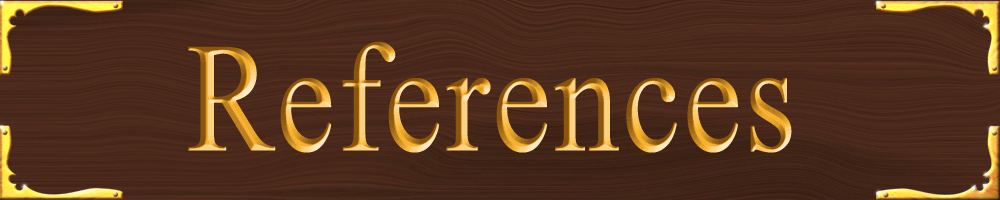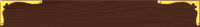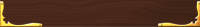Physics Reference Tables

## Greek Alphabet- used as variables or symbols

Many letters represent specific values or measurements

 Use in Physics Capital Name Lower Case Use in Physics Α Alpha α angle Β Beta β angle torque Γ Gamma γ E/mc2, angle change Δ Delta δ small change Ε Epsilon ε electromotive force Ζ Zeta ζ Η Eta η index of refraction angle Θ Theta θ most common symbol for angle Ι Iota ι rarely used- looks too much like i Κ Kappa κ rate, lambda baryon Λ Lambda λ wavelength, rate Μ Mu μ coefficient of friction, muon, micro- Ν Nu ν frequency, nutrino charmed baryon Ξ Xi ξ Ο Omicron ο rarely used- looks like 0 product operator Π Pi π Hello! Pi! also a pion Ρ Rho ρ density, resistivity sum of Σ Sigma σ conductivity, cross section, area density Τ Tau τ mean lifetime, tau lepton Υ Upsilon υ rearely used- look slike v wave function, angle Φ Phi φ wave function, angle Χ Chi χ wave function Ψ Psi ψ wave function Ω Omega ω angular frequency## Alphabet- used as variables or symbols

 Use in Physics Capital Lower Case Use in Physics atomic mass A a acceleration magnetic flux density, binding energy B b capacitance C c speed of light in a vaccuum electric displacement D d distance energy, electric field strength E e eccentricity force F f friction universal gravitational constant G g acceleration due to gravity, gravitational field strength magnetic field strength H h Plank's constant electric current, moment of inertia I i initial value, imaginary number current density J j impulse kinetic energy K k spring constant luminosity, inductance L l length magnetization, moment M m mass normal vector, atomis number N n number, refraction index not used- looks too much like zero O o not used- looks too much like zero power, pressure, polarization P p momentum heat Q q charge resistance, radiancy R r distance (radius), position S s arc length temperature T t time potential energy U u relativistic mass, energy density voltage, potential V v velocity W w weight X x position Y y impedance, proton number Z z red shiftMedina On-Line
Since 1994
Email: mrsciguy@optonline.net